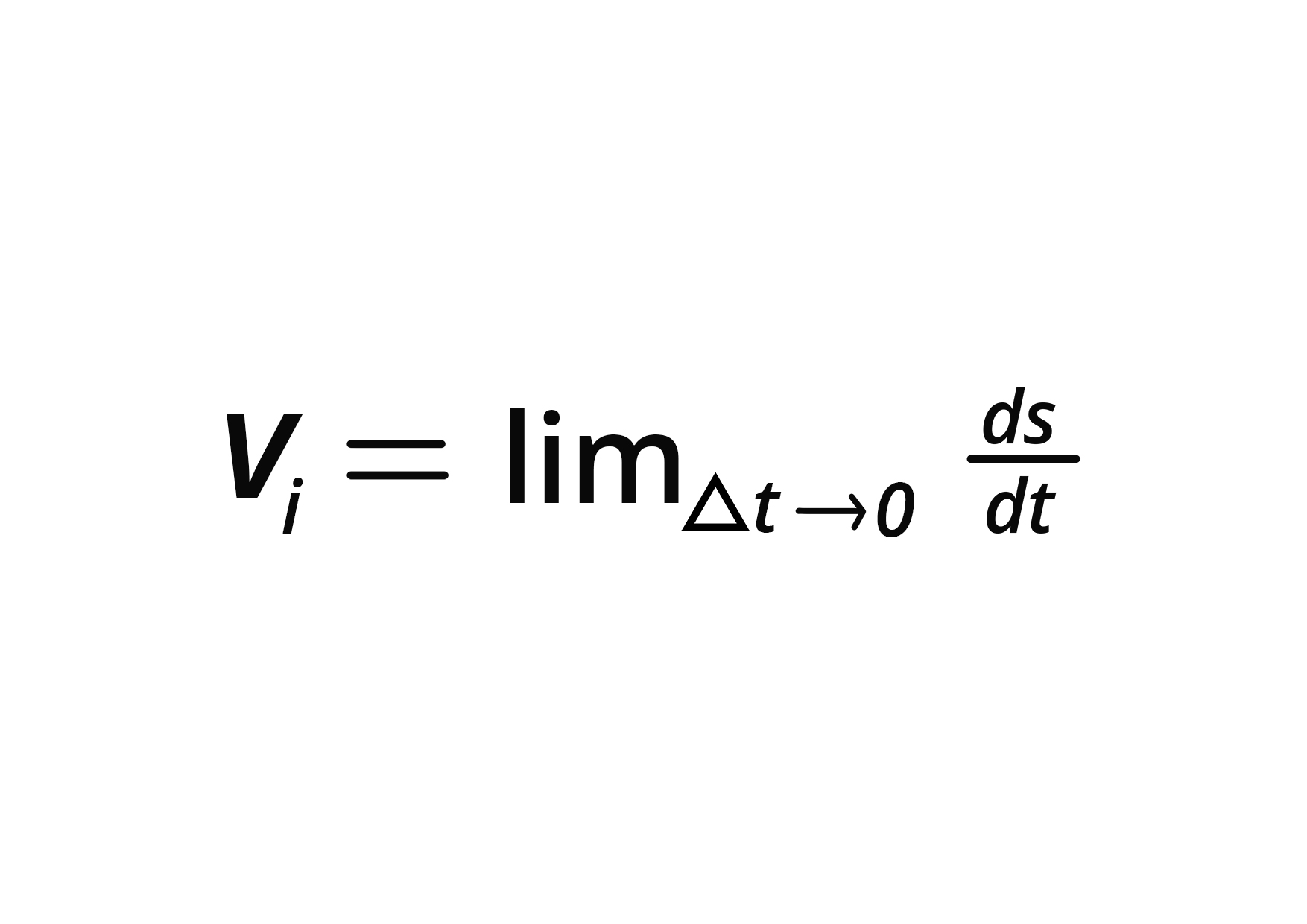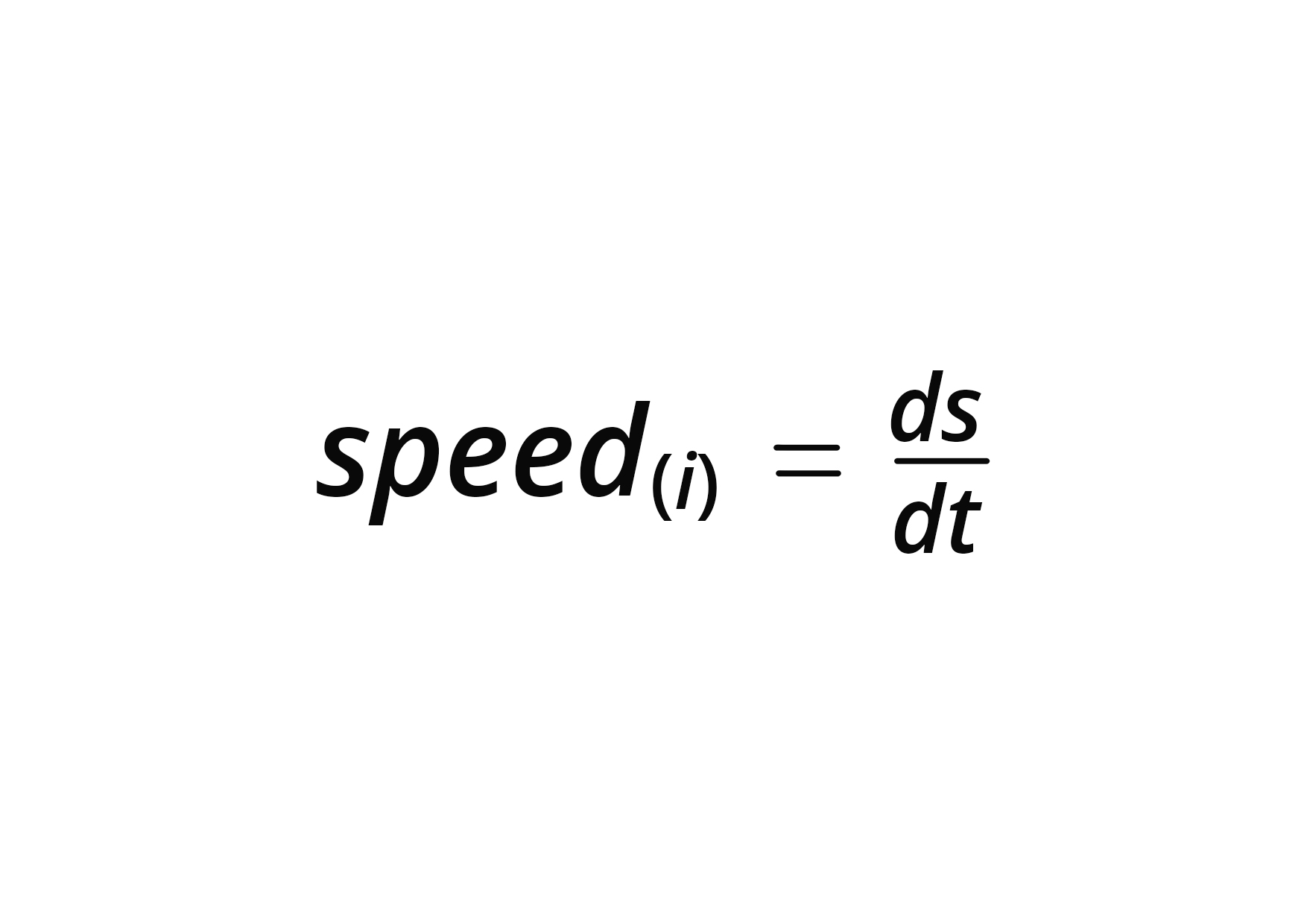• Call Now

1800-102-2727•

# Instantaneous Speed and Velocity - Physics

## Instantaneous Velocity

The rate of growth of location over a very short time frame is characterized as instantaneous velocity (almost zero). m/s is the SI unit of speed. The amount of the immediate velocity is known as instantaneous speed. Its value is identical to those of instantaneous velocity, even though it has no directions.

Simply said, the velocity of an item at a given point in time. The meaning of instantaneous velocity is the velocity of an object in motion at a certain point in time.The velocity profile of an item with uniform velocity could be the same just like its standard velocity.

It's calculated in the same way as average velocity, but with a shorter period of time. We know because total displacement based on total time equals average velocity for a particular time span. The movement approaches 0 as the time window approaches zero. However, the ratio of movement to time has a non-zero limit, which is known as instantaneous velocity.

The equation for instantaneous velocity is

Vi=lim∆t→0ds/dt

Where,
The small-time interval is denoted by the Δt.
The instantaneous velocity is Vi.
The displacement is denoted by the letter s.
Time is denoted by t.

m/s is the SI unit for instantaneous velocity. It is a vector quantity. The slope of the distance-time graph, commonly known as the x-t graph, may also be used to determine it.

## Instantaneous Speed

The distance travelled divided by the total time required equals the average speed for a given time span. The distance travelled approaches 0 as the time window approaches zero. However, the instantaneous speed is the non-zero limit of the distance-to-time ratio. We could also say that instantaneous speed at any given moment is the amount of instantaneous velocity at a certain time, to put it another way.

The formula for instantaneous speed is -

Speed(i)=ds/dt

Where,
The distance is denoted by ds.
The time interval is denoted by dt.
The instantaneous speed is speed(i).

m/s is the SI unit for instantaneous speed. It is a scalar quantity. The instantaneous velocity may be either linear or angular.

The difference between instantaneous speed and instantaneous velocity is given below -

 Parameter Instantaneous Speed Instantaneous Velocity Definition It is the magnitude of instant velocity at a given instant of time It is the change of position that takes place at a very small interval of time Quantity metrics It is a scalar quantity It is a vector quantity Formulation Speed(i)=ds/dt Vi=lim∆t→0ds/dt Unit of measurement Meters per second Meters per second

## Questions on Instantaneous Speed and Instantaneous Velocity

• Describe the concept of instantaneous velocity.

The speed of a moving item at a given point in time.

• Write the instantaneous velocity units.The units of instantaneous velocity are meters per second (m/s).

• Is instantaneous velocity a scalar or a vector?

Instantaneous velocity is a vector quantity, thus it's a vector quantity.

• Define the pace at which something happens right now.

The object's velocity at a specific moment or instant in time.

• If the item is traveling at a speed of 40 miles per hour. What is the instantaneous speed of the object?

The object's immediate speed is 40 miles per hour.

• What is the relationship between instantaneous velocity and instantaneous speed?

The quantity of the instantaneous velocity is called Instantaneous Speed.Talk to our expert
Resend OTP Timer =
By submitting up, I agree to receive all the Whatsapp communication on my registered number and Aakash terms and conditions and privacy policy